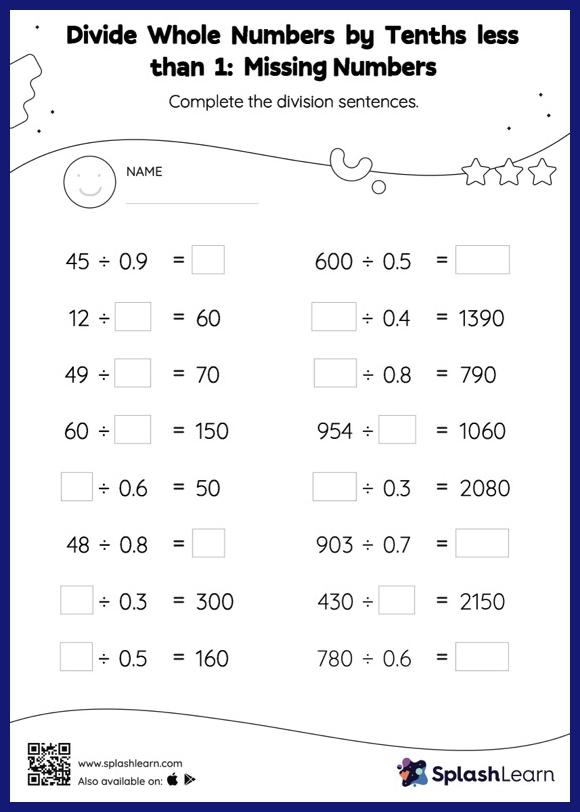# Divide Whole Numbers by Tenths less than 1 without Remainder: Missing Numbers Worksheet

Home > Divide Whole Numbers by Tenths less than 1 without Remainder: Missing NumbersDivide whole numbers by tenths less than 1 without remainder worksheet is a great way to help learners become fluent with the concept of division. When dividing a whole number by a decimal, students divide without considering the decimal point and then move each digit of the quotient to the left based on the number of decimal digits in the divisor. Students utilize this concept to find the missing number in divide whole numbers by tenths less than 1 without remainder worksheet. In each problem, the numbers are laid out in the horizontal format. Students should try to use different strategies involving composing and decomposing numbers to solve these problems. This will help them develop flexibility and fluency.